Published 06.02.2019 в 12:00 | Guide rating: 248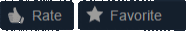### Notes

As more insignia come out I will update the Guide.
Please, if you find any errors, please let me know, my English is far from perfect.
Don't forget to press RATE and FAVORITE :]### Introduction

Since I first met Steam, I asked myself who were the people who had the most games on the platform, how many Steam games were in the catalog, etc.
, how can I check out this topic I opened in 2014: "Qual é o brasileiro que tem mais jogos e DLCs na Steam?".
This curiosity took me a while to research and found that Steam had a lot more games than I had imagined.
This Guide is the conclusion of my curiosity.
I want to keep the Guide up to date with reference sources whenever new information appears.
Almost everything on this subject is in English and I decided to write this Guide also in English to have a greater reach, unfortunately I am not very fluent in that language and most probably some errors will appear.
If you can, leave corrections and tips for new information in the comments.
DLC's, F2P games, etc.
, does not count.
The variable G below is for the number of games that one wants to calculate according to the badge level.
Originally posted by Baken: PLEASE keep in mind that since you cannot have fractions of a point of XP, the XP is rounded.
If Valve is truncating instead of rounding, well, it would probably make the equations a bit more elegant or something, but this is enough work as it stands.
I'm also basing this off the assumption that the constant in the equation for each badge is a whole number, which I think may be reasonable for a programming point of view.

### Table of the collectors' game insignia

Insignia Name Number of games Minimum XP XP Calculation One-Stop Shopper 1 to 4 100 XP XP = 94 + (6 x G) Selected Collector 5 to 9 125 XP XP = 100 + (5 x G) Adept Accumulator 10 to 24 150 XP XP = 150 + (3.
31 x (G - 10)) Sharp-Eyed Stockpiler 25 to 49 200 XP XP = 150 + (2 x G) Collection Agent 50 to 99 250 XP XP = 250 + (1.
49 x (G - 50)) Power Player 100 to 249 325 XP XP = 325 + (1.
1585 x (G-100)) Game Mechanic 250 to 499 500 XP XP = 250 + G Director of Acquisitions 500 to 999 750 XP XP = 250 + G Game Industry Guardian 1000 to 1999 1250 XP XP = 250 + G Gaming God 2000 to 2999 2250 XP XP = 250 + G Accrual Expert 3000 to 3999 3250 XP XP = 250 + G Ambassador of Amassment 4000 to 4999 4250 XP XP = 250 + G Digital Deity 5000 to 5999 5250 XP XP = 250 + G Collection King 6000 to 6999 6250 XP XP = 250 + G Magnate of Amassment 7000 to 7999 7250 XP XP = 250 + G Stockpiler Supreme 8000 to 8999 8250 XP XP = 250 + G Almighty Aggregator 9000 to 9999 9250 XP XP = 250 + G Master Gatherer 10000 to 10999 10250 XP XP = 250 + G Omnipotent Assemblert 11000 to 11999 11250 XP XP = 250 + G Acquisition Idol 12000 to 12999 12250 XP XP = 250 + G Game Collector: 13,000+ 13000 to 13999 13250 XP XP = 250 + G Game Collector: 14,000+ 14000 to 14999 14250 XP XP = 250 + G Game Collector: 15.
000+ 15000 to 15999 15250 XP XP = 250 + G Game Collector: 16.
000+ 16000 to 16999 16250 XP XP = 250 + G Game Collector: 17.
000+ 17000 to 17999 17250 XP XP = 250 + G Game Collector: 18.
000+ 18000 to 18999 18250 XP XP = 250 + G Game Collector: 19.
000+ 19000 to 19999 19250 XP XP = 250 + G Game Collector: 20.
000+ 20000 to 20999 20250 XP XP = 250 + G Game Collector: 21.
000+ 21000 to 21999 21250 XP XP = 250 + G Game Collector: 22.
000+ 22000 to 22999 22250 XP XP = 250 + G Game Collector: 23.
000+ 23000 to 23999 23250 XP XP = 250 + G Game Collector: 24.
000+ 24000 to 24999 24250 XP XP = 250 + G Game Collector: 25.
000+ 25000 to 25999 25250 XP XP = 250 + G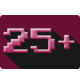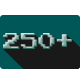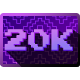### About formula to discover XP

Badge level Account example 1+ One-Stop Shopper XP = 94 + (6 x G) XP = 94 + (6 x 2) XP = 94 + 12 XP = 106 5+ Selected Collector XP = 100 + (5 x G) XP = 100 + (5 x 7) XP = 100 + 35 XP = 135 10+ Adept Accumulator XP = 150 + (3,31 x (G - 10)) XP = 150 + (3,31 x (23 - 10)) XP = 150 + (3,31 x 13) XP = 150 + 43,03 XP = 193,03 25+ Sharp-Eyed Stockpiler XP = 150 + (2 x G) XP = 150 + (2 X 42) XP = 150 + 84 XP = 234 50+ Collection Agent XP = 250 + (1.
49 x (G - 50)) XP = 250 + (1,49 x (80-50)) XP = 250 + (1,49 x 30) XP = 250 + 44,7 XP = 294,7 100+ Power Player XP = 325 + (1.
1585 x (G-100)) XP = 325 + (1.
1585 x (173 - 100)) XP = 325 + (1.
1585 x 73) XP = 325 + 84,5705 XP = 409,5705 250+ Game Mechanic XP = 250 + G XP = 250 + 486 XP = 736 500+ Director of Acquisitions XP = 250 + G XP = 250 + 550 XP = 800 1K+ Game Industry Guardian XP = 250 + G XP = 250 + 1606 XP = 1856 2K+ Gaming God XP = 250 + G XP = 250 + 2232 XP = 2482 3K+ Accrual Expert XP = 250 + G XP = 250 + 3335 XP = 3585 4K+ Ambassador of Amassment XP = 250 + G XP = 250 + 4300 XP = 4550 5K+ Digital Deity XP = 250 + G XP = 250 + 5765 XP = 6015 6K+ Collection King XP = 250 + G XP = 250 + 6917 XP = 7167 7K+ Magnate of Amassment XP = 250 + G XP = 250 + 7522 XP = 7772 8K+ Stockpiler Supreme XP = 250 + G XP = 250 + 8049 XP = 8299 9K+ Almighty Aggregator XP = 250 + G XP = 250 + 9265 XP = 9515 10K Master Gatherer XP = 250 + G XP = 250 + 10181 XP = 10431 11K Omnipotent Assemblert XP = 250 + G XP = 250 + 11939 XP = 12189 12K Acquisition Idol XP = 250 + G XP = 250 + 12976 XP = 13226 13K Game Collector: 13,000+ XP = 250 + G XP = 250 + 13198 XP = 13448 14K Game Collector: 14,000+ XP = 250 + G XP = 250 + 14779 XP = 15029 15K Game Collector: 15,000+ XP = 250 + G XP = 250 + 15486 XP = 15736 16K Game Collector: 16,000+ XP = 250 + G XP = 250 + 16513 XP = 16763 17K Game Collector: 17,000+ XP = 250 + G XP = 250 + 17681 XP = 17931 18K Game Collector: 18,000+ XP = 250 + G XP = 250 + 18000 XP = 18250 19K Game Collector: 19,000+ XP = 250 + G XP = 250 + 19035 XP = 19285 20K Game Collector: 20,000+ XP = 250 + G XP = 250 + 20822 XP = 21072 21K Game Collector: 21,000+ XP = 250 + G XP = 250 + 21923 XP = 22173 22K Game Collector: 22,000+ XP = 250 + G XP = 250 + 22111 XP = 22361 * I can not find anyone with game collector badge level 23, 24, and 25 for me to print and illustrate the account example.
Probably no one has games over 22K.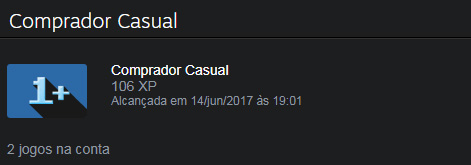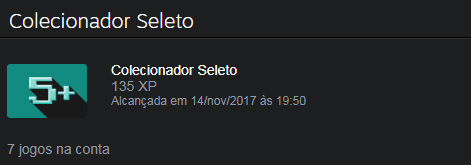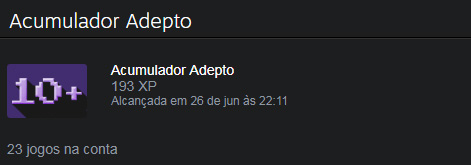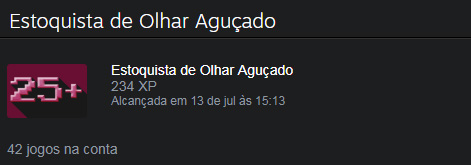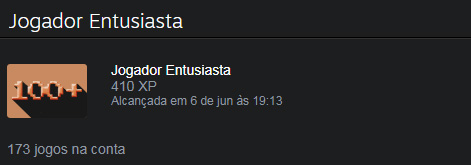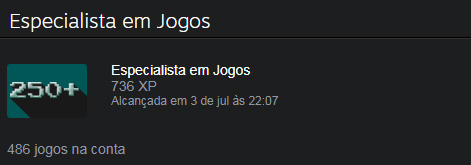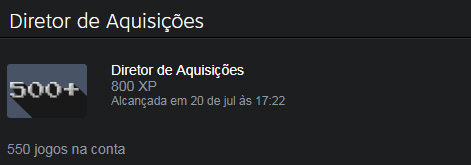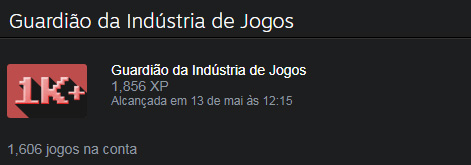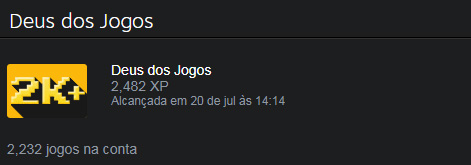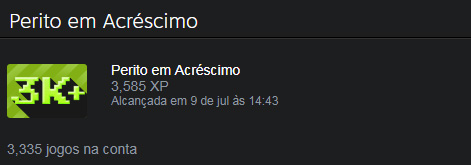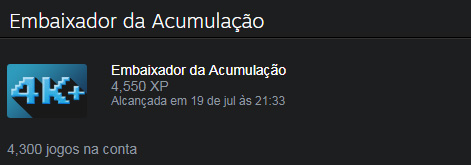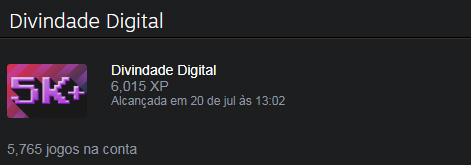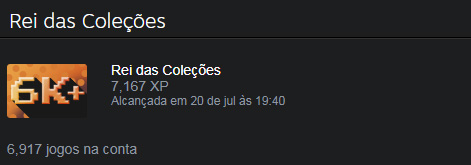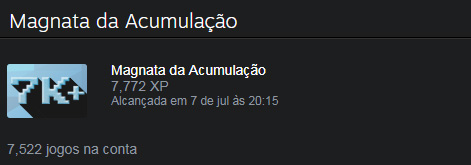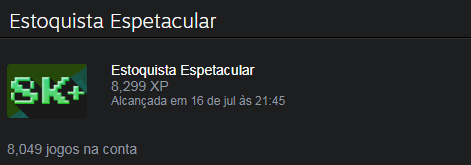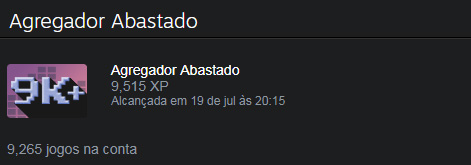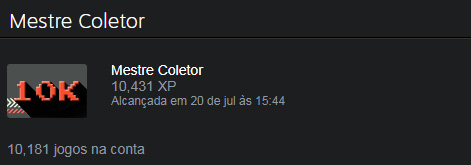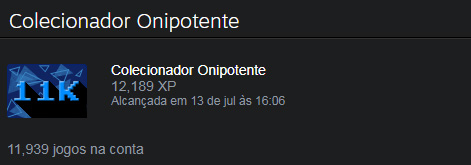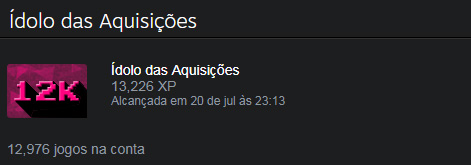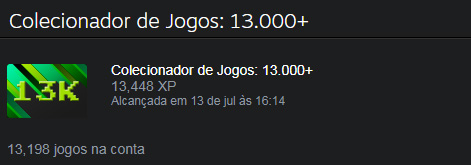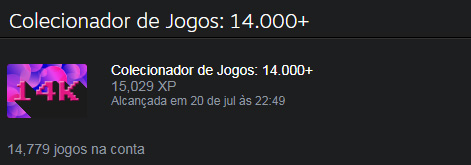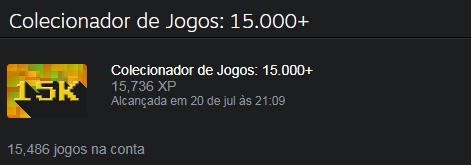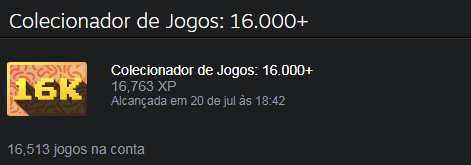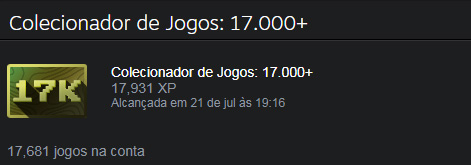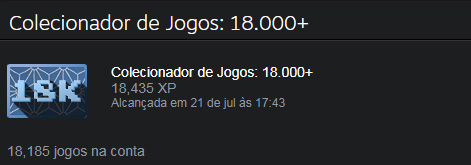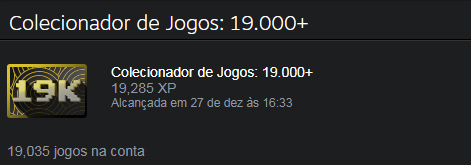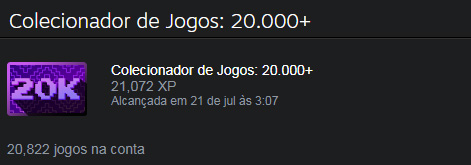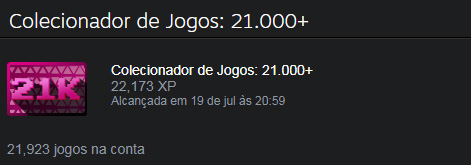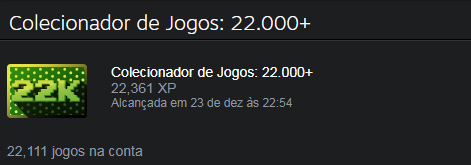Ylvox.
(2018).